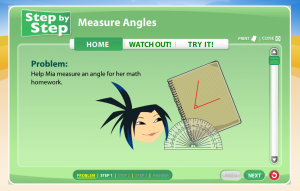# Understanding Angles and Concepts of Angle Measurement

##Measurement & Data – 4th Grade

#### Geometric measurement: understand concepts of angle and measure angles.

CCSS.Math.Content.4.MD.C.5
Recognize angles as geometric shapes that are formed wherever two rays share a common endpoint, and understand concepts of angle measurement:
CCSS.Math.Content.4.MD.C.5.a
An angle is measured with reference to a circle with its center at the common endpoint of the rays, by considering the fraction of the circular arc between the points where the two rays intersect the circle. An angle that turns through 1/360 of a circle is called a "one-degree angle," and can be used to measure angles.
CCSS.Math.Content.4.MD.C.5.b
An angle that turns through n one-degree angles is said to have an angle measure of n degrees.

Teacher Notes
An angle is a geometric figure formed when two rays share the same endpoint. Angles within a circle can be measured by turning the angle through the 360 degrees of the circle.
Some students can draw from their prior knowledge of hearing the terms 360 or 180 in skateboarding.
One degree angles are angles that open only one degree. Larger angles are created by a series of one degree angles and can be named for their size.

Student Knowledge Goals
I know an angle is the union of two rays with the same initial point.
I know angles are measured with reference to a circle with its center at a common endpoint of the rays.
I know the unit of measure for angles is degrees.
I know a full rotation from the center of a circle is 360 degrees.
I know an angle that turns through 1/360 degree is called a "one degree" angle.
I know that "one degree" angle can be used to measure angles.
I can measure an angle using a protractor.
I can sketch angles when given a measurement.
I can use a protractor to create a give angle.

I know an angle is the union of two rays with the same initial point.
I know angles are measured with reference to a circle with its center at a common endpoint of the rays.
I know an angle that turns counterclockwise through "n" one-degree angles has a measure of "n" degrees

Vocabulary
arc
central angle
circular
degree
endpoint
line segment
point
ray
turn
vertex

Lessons
Engage NY Module 3 A-1 – Identify and draw points, lines, line segments, rays, and angles and recognize them in various contexts and familiar figures.
Engage NY Module 3 A-2 – Use right angles to determine whether angles are equal to, greater than, or less than right angles.  Draw right, obtuse, and acute angles.
Engage NY Module 3 A-3 – Identify, define, and draw perpendicular lines.
Engage NY Module 3 A-4 – Identify, define, and draw parallel lines.
Engage NY Module 3 B-5 – Use a circular protractor to understand a 1-degree angle as 1/360 of a turn.  Explore benchmark angles using the protractor.
Engage NY Module 3 B-6 – Use varied protractors to distinguish angle measure from length measurement.
Measuring Angles

Student Video Lessons
Virtual Nerd – Angles
Virtual Nerd – Degrees
Study Jams – Review: Types of Lines
Study Jams – Review: Classify Angles
Study Jams – Construct Angles
Study Jams – Measuring Angles

Online Problems and Assessments
Khan Academy – Questions and Video Lessons
Angles of 90, 180, 270, and 360 degrees
Angles of 90, 180, 270, and 360 degrees
Estimate angle measurements